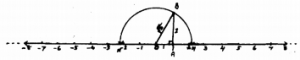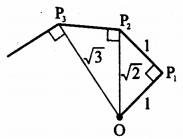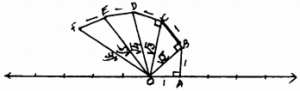# KSEEB Solutions for Class 9 Maths Chapter 1 Number Systems Ex 1.2

In this chapter, we provide KSEEB SSLC Class 9 Maths Chapter 1 Number Systems Ex 1.2 for English medium students, Which will very helpful for every student in their exams. Students can download the latest KSEEB SSLC Class 9 Maths Chapter 1 Number Systems Ex 1.2 pdf, free KSEEB SSLC Class 9 Maths Chapter 1 Number Systems Ex 1.2 pdf download. Now you will get step by step solution to each question.

## Karnataka Board Class 9 Maths Chapter 1 Number Systems Ex 1.2

Question 1.
State whether the following statements are true or false. Justify your answers.
(i) Every irrational number is a real number.
True. Because set of real numbers contain both rational and irrational number.

(ii) Every point on the number line is of the form m−−√. where’m’ is a natural number.
False. Value of m−−√ is not netagive number.

(iii) Every real number is an irrational number.
False. Because set of real numbers contain both rational and irrational numbers. But 2 is a rational number but not irrational number.

Question 2.
Are the square roots of all positive integers irrational? If not, give an example of the square root of a number that is a rationed number.
Square root of all positive integers is not an irrational number.
E.g. 4–√ = 2 Rational number.
9–√ = 3 Rational number.

Question 3.
Show how 5–√ can be represented on the number line.
5–√ can be represented on number line:In the Right angled ∆OAB ∠OAB = 90°.
OA = 1 cm, AB = 2 cm., then
As per Pythagoras theorem,
OB2 =OA2 + AB2
= (1)2 + (2)2
= 1 + 4
OB2 = 5
∴ OB = 5–√
If we draw semicircles with radius OB with ‘O’ as centre, value of 5–√ on number line
5–√ = OM = +2.3
and 5–√ = ON = -2.3 (accurately).

Question 4.
Classroom activity (Constructing the ‘square root spiral’): Take a large sheet of paper and construct the ‘square root spiral’ in the following fashion.Start with a point O and draw a line segment OP1 of unit length. Draw a line segment P1P22 perpendicular to OP1 of unit length (see fig.). Now draw a line segment P2P3 perpendicular to OP2. Then draw a line segment P3P4 perpendicular to OP3. Continuing in this manner, you can get the line segment Pn-1Pn by drawing a line segment of unit length perpendicular to OPn-1. In this manner, you will have created the points P2, P3, …………….. pn, …………… and joined them to create a beautiful spiral depicting 2–√⋅3–√,4–√,……
Classroom activity :i) OA = 1 Unit, AB = 1 Unit, ∠A = 90°,
∴OB2 = OA2 + AB2
= (1)2 + (1)2
= 1 + 1
OB2 = 2
∴OB = 2–√
Similarly, square root spiral can be continued.

All Chapter KSEEB Solutions For Class 9 Maths

—————————————————————————–

All Subject KSEEB Solutions For Class 9

*************************************************

I think you got complete solutions for this chapter. If You have any queries regarding this chapter, please comment on the below section our subject teacher will answer you. We tried our best to give complete solutions so you got good marks in your exam.

If these solutions have helped you, you can also share kseebsolutionsfor.com to your friends.

Best of Luck!!